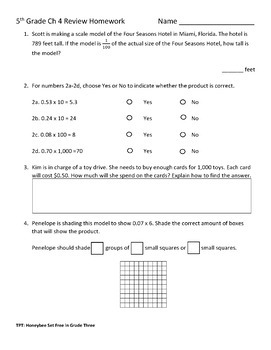# Fifth Grade Go Math Chapters 1-11 Review Homework BUNDLESubject
Resource Type
Format
Zip (20 MB)
Standards
\$25.00
Bundle
List Price:
\$33.00
You Save:
\$8.00
\$25.00
Bundle
List Price:
\$33.00
You Save:
\$8.00

#### Products in this Bundle (11)

showing 1-5 of 11 products

#### Also included in

1. This is a bundle for ALL Kindergarten-5th Grade Go Math! chapter resources I have created. These math reviews are correlated to the Go Math! series. This bundle is geared towards those that teach math to multiple grade levels, switch grade levels frequently, or need to differentiate math due to vari
\$150.00
\$213.00
Save \$63.00

### Description

This is a bundle that includes all 11 fifth grade Go Math! chapter review assignments. Each review can be used as a homework assignment, an in class review, or another form of the fifth grade chapter test. Each chapter review includes the same amount of questions as the review/test from the book, as well as, the chapter test. Each chapter review includes an answer key.

Total Pages
N/A
Included
Teaching Duration
N/A
Report this Resource to TpT
Reported resources will be reviewed by our team. Report this resource to let us know if this resource violates TpT’s content guidelines.

### Standards

to see state-specific standards (only available in the US).
Classify two-dimensional figures in a hierarchy based on properties.
Understand that attributes belonging to a category of two-dimensional figures also belong to all subcategories of that category. For example, all rectangles have four right angles and squares are rectangles, so all squares have four right angles.
Represent real world and mathematical problems by graphing points in the first quadrant of the coordinate plane, and interpret coordinate values of points in the context of the situation.
Use a pair of perpendicular number lines, called axes, to define a coordinate system, with the intersection of the lines (the origin) arranged to coincide with the 0 on each line and a given point in the plane located by using an ordered pair of numbers, called its coordinates. Understand that the first number indicates how far to travel from the origin in the direction of one axis, and the second number indicates how far to travel in the direction of the second axis, with the convention that the names of the two axes and the coordinates correspond (e.g., 𝘹-axis and 𝘹-coordinate, 𝘺-axis and 𝘺-coordinate).
Solve real world problems involving division of unit fractions by non-zero whole numbers and division of whole numbers by unit fractions, e.g., by using visual fraction models and equations to represent the problem. For example, how much chocolate will each person get if 3 people share 1/2 lb of chocolate equally? How many 1/3-cup servings are in 2 cups of raisins?